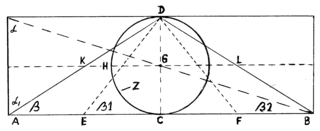## Sunday, October 13, 2013

### PRECESSION OF THE EQUINOX

The base length of the Great Pyramid: 23,192.867 cm

The Pyramid’s Code: 1 day = 2.466586266 cm

1000 x 23,192.867 cm = 23,192,867 cm

23,192,867 cm = 9,402,820.132 days = 25,744.08237 years =  circular movement of the Earth's axis or precession of the equinoxes (annual motion is about  0.013983796° =  50.3416661 seconds of arc per year, or 1 degree every 71.5113407 years.

## Friday, October 4, 2013

### Geometrical scheme of the UniverseGeometrical scheme of the Universe
.
DC = diameter of the circle Z =
GD = radius of the circle Z =
Angle β1 = Pyramid’s ascent = 51,85399754°
Tangent β1 = 1,273240621
CircumferenceC = (d/tang. β1) x 4
AreaA = (r²/tang. β1) x 4
Angle β = ascent of the King’s Chamber north channel = 32,48165854°
Tangent β = 0,63662031
Circumference(d/tang. β) x 2
Area
(r²/tang. β) x 2
Angle β2 = 17,65680115°
Tangent β2 = 0,318310155 = 1 : 3,14159
CircumferenceC = d/tang. β2 = AB
AreaA = r²/tang. β2

DH = r/tangβ KG = GL = AE EC = CF = FB = 1/4 AB
DC = D/tang. β = AC = CB = 1/2 AB

Tangent of the angle α (Alpha) = 3,14159 = Pi
Tangent of the angle Alpha= 1,570795 = Pi/2

The rectangle’s Area = Area of the sphere around the circle Z

## Sunday, September 29, 2013

### Why was the Great Pyramid of Giza built

Why was the Great Pyramid of Giza built? The Great Pyramid is a time capsule  which holds ancient wisdom, knowledge about the ancient and modern metrology, knowledge about the size of the Earth, and fundamental but important knowledge about astronomy, with an exact precision which is only in our time established by the modern  astronomical science. This is the knowledge about our material  physical world.
The numbers and the measurements of the  Great Pyramid also hold and show the knowledge of future events and show the major historical moments of human history: creation of the world, the time when the Pyramid was built, the great biblical flood, the birth of the Messiah, His suffering, resurrection and his second coming to Earth. This is the knowledge about our spiritual world.